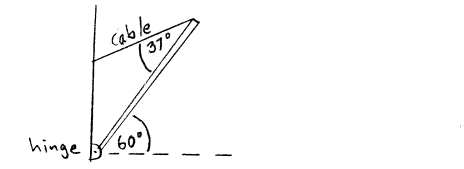# Problem: A uniform bar (I = 1/3 ML2 for an axis at one end) has mass M = 5.00 kg and length L = 6.00 m. The lower end of the bar is attached to the wall by a frictionless hinge. The bar is held stationary at an angle of 60° above the horizontal by a cable that runs from the upper end of the bar to the wall. The cable makes an angle of 37° with the bar. For an axis at the hinge, what is the torque due to the weight of the bar?

###### FREE Expert Solution
94% (182 ratings)
###### Problem Details

A uniform bar (I = 1/3 MLfor an axis at one end) has mass M = 5.00 kg and length L = 6.00 m. The lower end of the bar is attached to the wall by a frictionless hinge. The bar is held stationary at an angle of 60° above the horizontal by a cable that runs from the upper end of the bar to the wall. The cable makes an angle of 37° with the bar. For an axis at the hinge, what is the torque due to the weight of the bar?